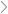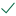Questions & AnswersJava ProgrammingQuestionAnswered step-by-step

# Equal Trees In this problem, your task is to complete  method...

Equal Trees In this problem, your task is to complete  method called equals that accepts another binary tree of integers as a parameter and compares the two trees to see whether they are equal to each other. If variables of type TreeIntSet called t1 and t2 have been initialized, then t1.equals(t2) will return true if the trees are equal and false otherwise. Notes and Constraints • Two empty trees are considered to be equal to each other. • Your method should be recursive

Full Trees In this , your task is to complete a method called isFull that returns true if a binary tree is full and false if it is not. A full binary tree is one in which every node has 0 or 2 children. Notes and Constraints • By definition, the empty tree is considered full. • Your method should be recursive.

Examples 1. If called on this tree, isFull returns false. 2. If called on this tree, isFull returns false. 3. If called on this tree, isFull returns true. (see image)

Complete methods here

/** A binary search tree based implementation of a set of integers.

*

*/

public class TreeIntSet {

private TreeNode root; // the overall root node of the tree

private int numElements; // the size of this set

/** A class for representing and manipulating individual tree nodes. */

private static class TreeNode {

/* Even though the following fields are declared public, this nested

* class is itself private. So only the methods inside the TreeIntSet

* class can actually instantiate TreeNode objects. */

public int value; // the data field

public TreeNode left; // reference to the left subtree

public TreeNode right; // reference to the right subtree

/** Constructs a new TreeNode with the specified datum value.

*

* Both children are set to null.

*

* @param value the value to be stored at this node.

*/

public TreeNode(int value) {

this.value = value;

this.left = null;

this.right = null;

}

}

/** Constructs a new empty tree. */

public TreeIntSet() {

this.root = null;

this.numElements = 0;

}

/** Returns a string representation of this tree.

*

* The elements are returned in ascending order, enclosed in curly braces.

*/

public String toString() {

if (this.numElements == 0)

return "{}";

// deal with the fencepost problem by trimming off the last ", " after

// the recursive helper completes

String repString = toString(this.root);

return "{" + repString.substring(0, repString.length()-2) + "}";

}

/** Returns the string representation of the tree rooted at the given node.

*

* Performs an in-order traversal of the supplied tree, so that elements

* are returned in ascending order.

*

* @param node the root of the tree to be traversed.

* @return a string representation of the tree rooted at node.

*/

private String toString(TreeNode node) {

if (node == null)

return "";

return (toString(node.left) + node.value + ", " + toString(node.right));

}

/** Inserts the supplied value into this set.

*

* @param value the value to be inserted into the set.

*/

}

/** Recursively inserts the supplied value into the BST rooted at node.

*

* @param node the root node of the (sub)tree into which we wish to insert

*             value.

* @param value the value to be inserted.

*/

private TreeNode add(TreeNode node, int value) {

if (node == null) {

node = new TreeNode(value);

this.numElements++; // update set size

}

else if (value < node.value)

else if (value > node.value)

// If value == node.value, then we don't insert value, because we are

// using the BST to implement a Set (that doesn't permit duplicates).

return node;

}

/** Returns the size of this set.

*

* @return the number of elements in this set.

*/

public int size() {

return this.numElements;

}

public boolean equals(TreeIntSet other) {

return true; // placeholder for compilation, remove when done

}

public boolean isFull() {

return true; // placeholder for compilation, remove when done

}

}

/** Tester program to verify the correctness of the TreeIntSet implementation.

*

*/

public class TreeIntSetClient {

public static void main(String[] args) {

TreeIntSet t1 = new TreeIntSet();

System.out.println(t1); // print out the elements in ascending order

System.out.println(t1.isFull()); // not a full tree

TreeIntSet t2 = new TreeIntSet();

System.out.println(t1.equals(t2)); // equal to the previous tree

System.out.println(t2.isFull()); // still not a full tree

}

}Solved by verified expert
``sectetur adipiscing elit. Nam lacinia pulvinar tortor nec facilisis. Pellentesque dapibus efficitur laoreet. Nam risus ante, dapibus a molestie consequat, ultrices ac magna. Fusce dui lectus, congue vel laoreet ac, dictum vitae odio. Donec aliquet. Lorem i``10,000 step-by-step explanations

## Step-by-step explanation

sectetu

``sectetur adipiscing elit. Nam lacinia p``

sectetur adipiscing elit. Nam lacinia pulvinar tortor nec facilisis. Pellentesque dapibus efficitur laoreet. Nam risus ante, dapibus a molestie consequat, ultrices ac magna. Fusce dui lectus, congue v

## Get unstuck with a CliffsNotes subscription